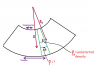# Magnetization in a rod bent into a torus

benf.stokes

## Homework Statement

I can't figure out why there is no bound current in the problem 6 (very subtle hint boldfaced) is the pdf below:
http://astronomy.mnstate.edu/cabanela/classes/phys370/homework/ps10.pdf [Broken]

Can anybody give me a hint as to why there should be no bound current

## The Attempt at a Solution

I know that the total dipole moment contained in a volume element must be constant. However i can't turn this into a relationship between the new M and the old one

Last edited by a moderator:

Homework Helper
Gold Member
See if you can follow the hint. How would the mass density ρ in the bent rod vary with radial distance s? See attached picture. Let point a be a point where the density happens to be the same as the unbent rod (ρo). Can you express the density ρ at an arbitrary point b in terms of the density ρo at a and the radial distances s and so?

If you can figure that out, then you should be able to see how the magnetization M varies with s.

#### Attachments

•Bent rod.png
5.5 KB · Views: 472
benf.stokes
I figured it out due to your help. Thanks :)